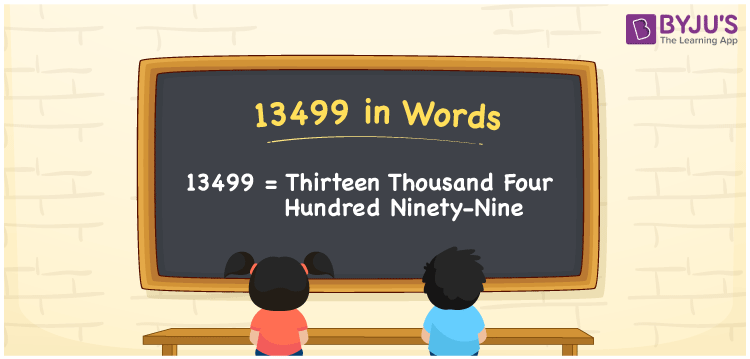# 13499 in Words

We can write 13499 in words as Thirteen thousand four hundred ninety-nine. If you paid Rs. 13499 in a shop, you could say, “I paid Thirteen thousand four hundred ninety-nine rupees at the shop”. That means we can communicate 13499 in words as Thirteen thousand four hundred ninety-nine. Let’s understand how to transform the number 13499 into word form using a place value chart here in this article.

 13499 in words Thirteen thousand four hundred ninety-nine Thirteen thousand four hundred ninety-nine in Numbers 13499

## 13499 in English Words

Generally, we use the English alphabet to express numbers in words. So, we can spell 13499 in English words as “Thirteen thousand four hundred ninety-nine”.## How to Write 13499 in Words?

In this section, you will learn how to convert the number 13499 into words with the help of a place value chart. So, create a five-column place value chart as 13499 is a five-digit number.

 Ten thousand Thousands Hundreds Tens Ones 1 3 4 9 9

Here, ones = 9, tens = 9, hundreds = 4, thousands = 3, ten thousand = 1

Let’s expand these digits as follows.

1 × Ten thousand + 3 × Thousand + 4 × Hundred + 9 × Ten + 9 × One

= 1 × 10000 + 3 × 1000 + 4 × 100 + 9 × 10 + 9 × 1

= 10000 + 3000 + 400 + 90 + 9

= Ten Thousand + Three thousand + Four hundred + Ninety + Nine

= Thirteen thousand + Four hundred ninety-nine {since ten + three = thirteen}

= Thirteen thousand four hundred ninety-nine

13499 is a natural number that is the successor of 13498 and predecessor of 13500.

13499 in words – Thirteen thousand four hundred ninety-nine

Is 13499 an odd number? – Yes

Is 13499 an even number? – No

Is 13499 a composite number? – No

Is 13499 a prime number? – Yes

Is 13499 a perfect square number? – No

Is 13499 a perfect cube number? – No

## Frequently Asked Questions on 13499 in Words

### How do you write 13499 in English words?

In English words, we can write the number 13499 as Thirteen thousand four hundred ninety-nine.

### How to write an amount of Rs. 13499 in words on a cheque?

On a cheque, we can write an amount of Rs. 13499 in words as “Thirteen thousand four hundred ninety-nine rupees only”.

### Express the sum 8400 + 5099 in words.

8400 + 5099 = 13499 The sum 8400 + 5099, i.e., 13499 can be expressed in words as Thirteen thousand four hundred ninety-nine.